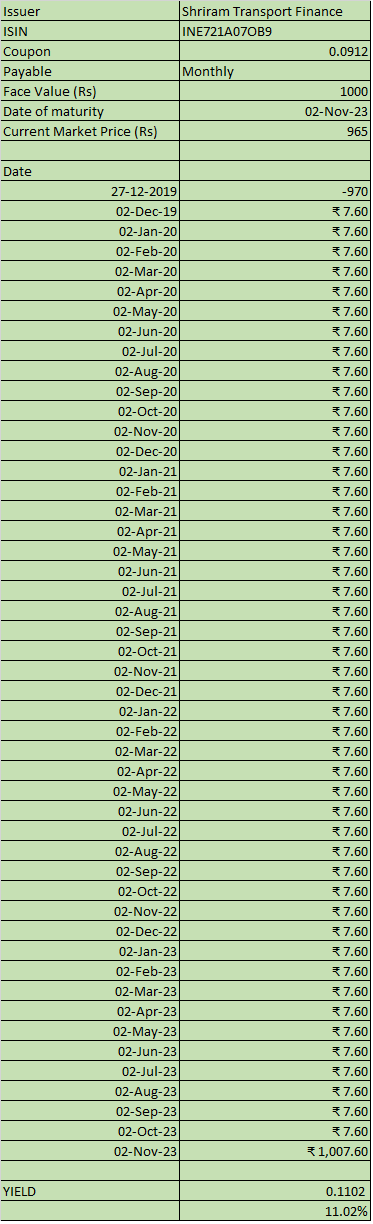# Explained: How to calculate yields on your bond investments

## You can calculate the yield using simple spreadsheet formulas

December 30, 2019 / 10:08 AM ISTTypically, we invest in bonds during their public issue. But, at times, we also consider bonds listed on the stock exchanges. The rate of interest (also known as the coupon rate) is an important criterion for investing. However, things are different when you buy bonds on the stock exchange. Although all listed (existing) bonds come with a published coupon rate, it is not the return that you would earn if you buy them.

Bond prices fluctuate. Depending on when you buy the bond or sell it, the return that you earn would be different from the interest rate that the instrument carries. The return you earn is called yield to maturity (YTM). It’s important to know your bond’s yield; here is how to calculate it.

Calculating yields

“YTM is the true indicator of return on investment.  Generally, investors calculate current yield by dividing the coupon by the price of the bond, which will not give the right picture, as the price includes the premium and accrued interest. And on maturity, an investor will get the face value and not the premium,” says Vikram Dalal, founder and managing director of Synergee Capital Services.

A bond quotes at a premium to the face value if there is a credit rating upgrade, fall in interest rates in the economy or positive investor perception. It can also quote at a discount if there is a rating downgrade, increase in interest rates, investor disinterest or lack of liquidity.

If a bond has a call option, which allows the issuer to redeem the bond early, you should calculate the yield to the date of call option as a prudent measure, assuming the bond will be called back.

Bonds are traded on the BSE and the NSE. You can access the details related to traded bonds from these links – BSE and NSE.

You can click on any specific issue and view the details. For example, Shriram Transport Finance’s NCD is a bond that offers a coupon of 9.12 per cent, payable monthly. The date of maturity is November 2, 2023.

Once you know this information, you need a spreadsheet (Microsoft Excel) to compute the yield on the bond.

Key in the data as shown in the screenshot below. The date column will specify the dates of the cashflows. The payout column mentions the absolute interest amount. On the date of maturity, the cash receipt will include the principal of Rs 1000 and the interest payout for that month.Now key in the formula of XIRR as shown below. XIRR used to calculate returns on investments made at different points of time. It is used to calculate returns when there are multiple cashflows – be they investments or redemptions spread over a period of time.In the above example, 10.67 per cent is the YTM for the bond. Since the bond has a monthly payout, the accrued interest part has been ignored. Otherwise, the yield would be a bit lower.

“Investors must take into account the accrued interest while calculating the YTM,” says Joydeep Sen, founder and CEO of wiseinvestor.in. In the case of annual interest payouts, this accrued interest needs to be considered. For example, in the case of a bond with face value of Rs 1000 and annual interest payout of 12 per cent, if you are computing the YTM at the end of the 10th month, the fair value of the security would stand at Rs 1100.

“When you use XIRR formula to compute the YTM, it assumes that the interim cashflows are re-invested at the yield,” says Joydeep. In reality, it may not be the case and in that scenario, the yield will be marginally lower.

Nikhil Walavalkar
first published: Dec 30, 2019 10:06 am